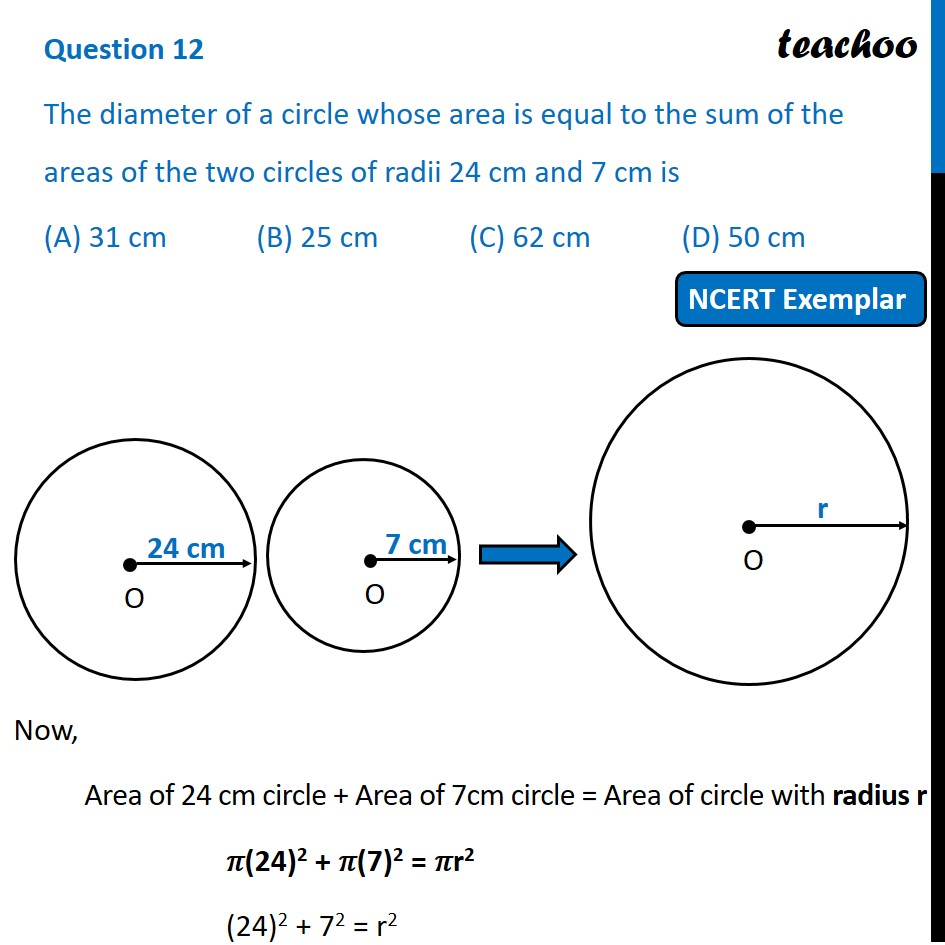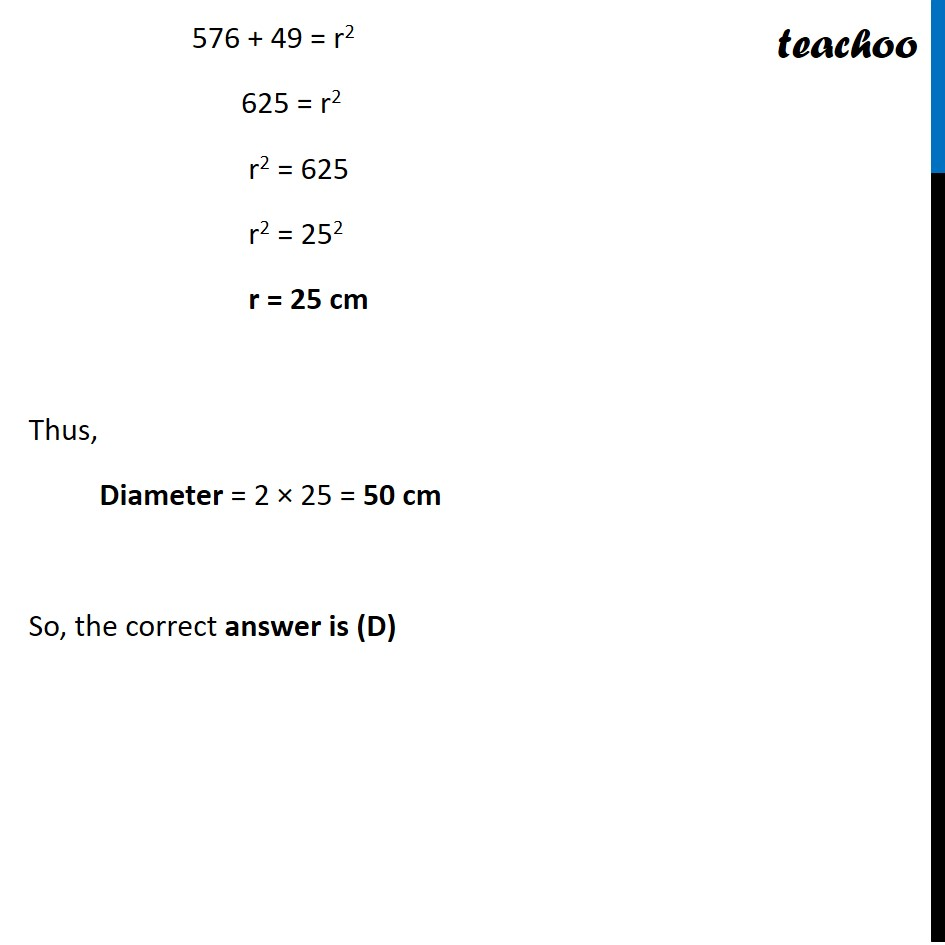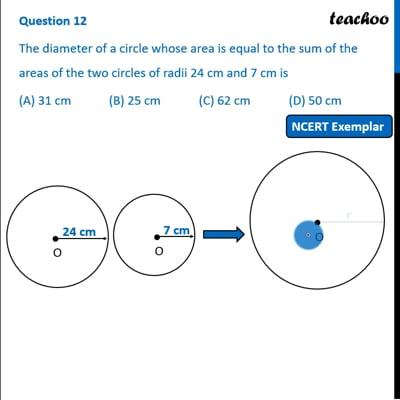NCERT Exemplar - MCQ

Chapter 12 Class 10 Areas related to Circles
Serial order wise

## (A) 31 cm   (B) 25 cm   (C) 62 cm   (D) 50 cmThis video is only available for Teachoo black users

Maths Crash Course - Live lectures + all videos + Real time Doubt solving!

### Transcript

Question 12 The diameter of a circle whose area is equal to the sum of the areas of the two circles of radii 24 cm and 7 cm is (A) 31 cm (B) 25 cm (C) 62 cm (D) 50 cm Now, Area of 24 cm circle + Area of 7cm circle = Area of circle with radius r 𝜋(24)2 + 𝜋(7)2 = 𝜋r2 (24)2 + 72 = r2 Now, Area of 24 cm circle + Area of 7cm circle = Area of circle with radius r 𝜋(24)2 + 𝜋(7)2 = 𝜋r2 (24)2 + 72 = r2 576 + 49 = r2 625 = r2 r2 = 625 r2 = 252 r = 25 cm Thus, Diameter = 2 × 25 = 50 cm So, the correct answer is (D)